Go back to  'PEMDAS'

While we know 1 and 1 is 2, how do we add larger numbers? This simple video will show you how adding larger numbers can be as simple as a snap of a finger!

Addition and subtraction are the most basic operations. These concepts are building blocks of understanding and operating on numbers. We add and subtract numbers, amounts, and values in our everyday lives. Children are mechanically introduced to these concepts using numbers. However, this may not be fruitful in the long run. Mechanically rote memorizing the procedures increases the chances of silly mistakes.Let’s say you go to the supermarket and pick 2 packets of tomatoes. One packet has 4 and the other has just one. Now, you put all the tomatoes together in one packet. How many tomatoes will that packet have? The packet will have 5 tomatoes. Putting together of quantities is nothing but an addition. To help your child visualize the operation, addition is putting two or more groups together to end up with a larger group in the end.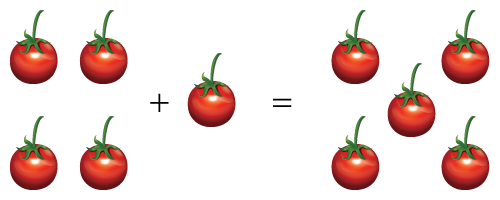When you get on your school bus, there are 6 children when you get up on the bus. So now there are 6 + 1 = 7 children on the bus. At the next stop, 5 more children get on the bus, and later two more children get on the bus. So, when the bus finally reaches your school, the number of children on the bus would be 6 + 1 + 5 + 2 = 14.

These are just a few examples showing how addition gets introduced at an early stage.

Addition can be visualised as 'putting together' or two or more quantities. It is important for children to see how when we put together two quantities it gives a larger group. For example, the image below shows, when the balls of the two groups were put together, it resulted in a third larger group.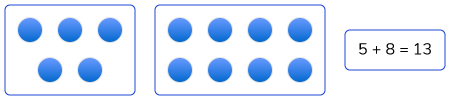Addition can be best introduced using real-life objects, and some visual models like 10-frame, number line, 20-frames. The smaller numbers make it easier to understand the concept. As you can see in the image given below there is a number line showing starting at 5 if you take 7 jumps then you will reach 12. Which is nothing but 5 + 7 = 12.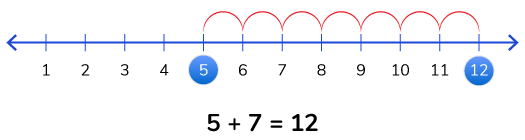Number line to show the addition of numbers: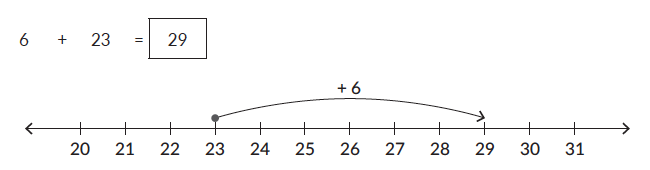Another simple idea used for the addition of two numbers is the concept of place value. As the numbers become bigger, they gain place values. The key concept to remember is that ONLY like places can be added at a time. So, if you have a four-digit number let's say1423, being added to a three-digit number let's say 176, the addition would look like as shown below. The digit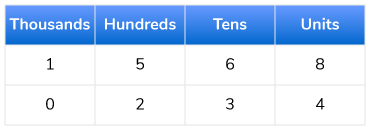So, in order to add 1423 and 176, you write both the numbers one below the other in such a way that the place values are all aligned. So, it would look like this:

\begin{align} {\rm{1}}\;\;{\rm{4}}\;\;{\rm{2}}\;\;{\rm{3}}\\ {\rm{ + }}\;\,{\rm{1}}\;\;{\rm{7}}\;\;{\rm{6}}\\ \overline {\underline {1\;\;5\;\;9\;\;9} } \end{align}

In ancient times, simple additions were done with the help of a simple, yet powerful instrument called the abacus.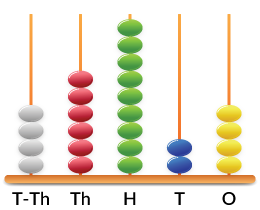## Topics closely related to Addition and Subtraction

The image shown below shows the topics one should be well versed with to understand addition & subtraction. It also shows the topics that these two operations are closely related to.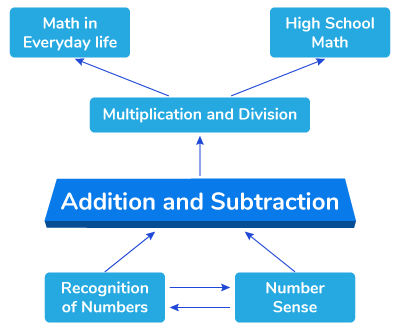Here are interesting videos to visualise addition. Know what happens when you carry while adding numbers.

Experience this in a Cuemath class. Book a Cuemath demo class with a nearby centre

## Concept of carryover/regrouping

In the above example, adding two digits result in a value less than 10. But what happens if the sum of a particular place value exceeds 10? We then carry over the value to the next higher place value. But why specifically 10? It is because we have a base-10 number system.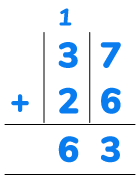In the image shown above the sum of 7 ones and 6 ones is 13. It is nothing but 1 ten and 3 ones. We retain the value of units place which is 3. And carry over 1 ten to the tens place. Thus adding 1 ten + 3 tens + 2 tens = 6 tens

Let's take another example to understand this better. Let's say we ahve to add 1476 + 246. The first step is to place the number under the right plae values. As shown below.

\begin{align} {\rm{1}}\;\;{\rm{4}}\;\;{\rm{7}}\;\;{\rm{6}}\\ {\rm{ + }}\;\,{\rm{2}}\;\;{\rm{4}}\;\;{\rm{6}}\\ \overline {\underline {\qquad\quad\;\;\,\\} } \end{align}

Now start adding the numbers from the ones place, if a value exceeds 10 then carryover to the next higher place value.

\begin{align} &\;\;\;\,{\;^1}\;\,{\;^1}\;\;\;\;\\ &{\rm{1}}\;\;{\rm{4}}\;\;{\rm{7}}\;\;{\rm{6}}\\ &{\rm{ + }}\;{\rm{2}}\;\;{\rm{4}}\;\;{\rm{6}}\\ &\overline {\underline {1\;\;7\;\;2\;\;2} } \end{align}

## How do you teach Addition?

1. Ask children to perform addition using real-life objects like pens, pencils, chocolates, marbles.

2. Use math manipulatives like colour counters, abacus, flats, rods and blocks to help children perform addition.

3. Pop quiz: you can ask children 5 rapid-fire questions on addition.

4. Based on the pocket money children receive Ask children to maintain a log of their expenses. At the end of the month, you can ask for the remaining amount.

5. Use flats, rods and blocks to show how the procedure works with larger numbers.

## Common mistakes or misconceptions

• In addition, when carrying over a number from one place value to the other, children make mistakes.
Generally, this happens because they have memorised the steps to add and if they forget the rules, mistakes happen. Instead of using the Base-10 blocks help children visualise the addition procedure. This way they’ll internalise the steps better.
• When adding a three-digit number to a two-digit number, students may place the numbers one below the other incorrectly.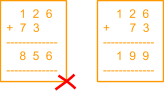This generally happens once again due to an incomplete understanding of place values and the idea of addition. Rather than telling students to simply align numbers from the right, it is better to ask them to model this addition problem using Base-10 blocks so they understand why the numbers are aligned from the right.

## Tips and Tricks

• Help children associate addition with common words used in everyday language like “put together”, “in all”, “altogether”, “total”, etc. This association will help them with comprehending word problems.
• Break numbers by place values to make addition easier. 32 + 46 can be split as 30 + 2 + 40 + 6. While this looks like it is more work, it makes mental addition easier. Help children add the tens first and then the ones.
Addition and Subtraction of two-digit numbers
Addition and Subtraction of two-digit numbers
Addition and subtraction of 3-digit numbers
Addition and subtraction of 3-digit numbers
Addition and Subtraction of 4-digit numbers
Addition and Subtraction of 4-digit numbers
Addition and Subtraction with numbers up to 20
Kg | Worksheet 3
Addition and Subtraction with numbers up to 20
Kg | Worksheet 4
Kg | Worksheet 2
Kg | Worksheet 1